American Journal of Nano Research and Applications
Volume 4, Issue 5, September 2016, Pages: 43-46

Enhancing Sensor Sensitivity Using Graphene-MTM Interface

R. J. El-Khozondar1, H. J. El-Khozondar2, M. M. Shabat3

1Physics Department, Al-Aqsa University, Gaza, Palestine

2Electrical Engineering Department, Islamic University, Gaza, Palestine

3Physics Department, Islamic University, Gaza, Palestine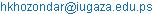(H. J. El-Khozondar)

R. J. El-Khozondar, H. J. El-Khozondar, M. M. Shabat. Enhancing Sensor Sensitivity Using Graphene-MTM Interface. American Journal of Nano Research and Applications. Vol. 4, No. 5, 2016, pp. 43-46. doi: 10.11648/j.nano.20160405.11

Received: August 11, 2016; Accepted: November 12, 2016; Published: January 17, 2017

Abstract: Sensitivity of a waveguide sensor consists of Graphene monolayer between metamaterials (MTMs) layer from below and dielectric layer from above is studied. In this work, we only considered Transverse Magnetic (TM) surface plasmon (SP) at terahertz (THz) range of frequency. Thus, Graphene is assumed to have constant surface conductivity with positive imaginary part. The homogenous sensitivity is derived from the dispersion equation and numerically calculated at different values of MTM parameter. Results show that this structure is valid as a very sensitive sensor that is capable of sensing small signals. Moreover, the sensitivity varies as MTM parameters varies at frequency ranges from 1-10 THz.

Keywords: Graphene, Metamaterials, Waveguide Sensor, Plasmon, TM, Sensitivity

Contents

1. Introduction

Waveguide sensors are considered as a main component in almost all branches of science including, biology, medicine and engineering. Thus, it become a big challenge to model sensors that can detect small changes in compact size. While light propagates through the waveguide, it could detect small changes at the cladding layer. In other words, waveguides are good candidates to design a sensor since they can transmit frequency in optical and microwave range depending on their structure [1-2]. Sensors might work as physical sensor if they transform a chemical or biological reaction in a measurable signal  and biochemical sensors that developed from slab structure with step index profile [4-5].

Terahertz (THz) radiation is used in astronomy and communication [6-7]. The propagation and detection of these waves require a development of a novel material such as Graphene and MTM. MTM is a manmade materials that has both negative permittivity and negative permeability [11-13]. Several researches have considered MTM in different applications including sensors , isolators , and transmission lines .

Graphene is a 2-D semiconductor material consisting of honeycomb atoms. It has optical and electronic properties that makes it very important in several applications at THz frequencies . It is mainly considered in many applications including antenna [8-10].

Many research have studied the propagation of plasmons along Graphene . At a certain range of frequencies, Graphene has a conductivity with a positive imaginary part which is needed to support plasmon in transverse magnetic fields modes (TM) . Graphene supports TM surface values with subwavelegth localization in the direction perpendicular to the surface. This property enhanced the idea of developing robust compact size devices .

In our work, we proposed a sensor consists of Graphene monolayer on top of Metamaterials (MTMs) layer and covered by dielectric layer. This work is an expansion to previous work for the authors in which they investigate the propagation of THz wave through waveguide has the same structure . In this work, we studied the possibility to use the same structure as a sensor to measure small changes at the cladding layer. We also would like to find out the effect of MTM parameters on the proposed structure keeping the product of the permittivity and permeability of MTM = 4 . The proposed waveguide structure is introduced in section 2. Section 3 presents the results of the proposed structure. The conclusion is given in section 4.

2. Theoretical Model

Fig. 1 shows the schematic of the proposed waveguide sensor structure. The proposed structure is a three layer waveguide. It consists of a Graphene layer surrounded from above by a dielectric layer and by a MTM from below. The dielectric has positive permeability ε1 and positive permeability μ1. MTM has both negative permeability ε2 and negative permeability μ2. The Graphene monolayer is characterized by a complex surface conductivity σ = σʹ + jσʹʹ, where σʹ is the real part and σʹʹ is an imaginary part. In this model, σ is considered to have positive imaginary part. Thus, Graphene can only support surface plasmons (SP) in Transvers Magnetic field (TM) .

When the imaginary part of Graphene conductivity is positive, the Graphene layer functions as a thin metal layer. The field components of TM modes are(1)

Where the propagation is assumed to be travelling along the z direction. Also, A1 and A2 are the field amplitudes to be find from the boundary conditions and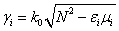with i=1 and 2, N is the effective refractive index and k0 is the wave number in free space.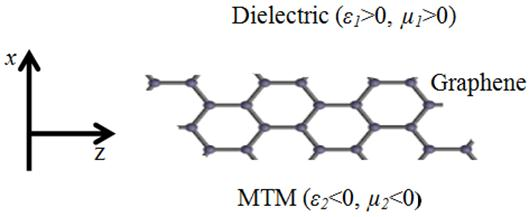Figure 1. The schematic of the dielectric-Graphene-MTM waveguide. The Graphene layer is at the interface between dielectric and MTM.

Using Maxwell's equation to obtain Ex and Ez and applying boundary conditions, the dispersion relation for TM modes can be written as(2)

The homogenous sensitivity (S) is calculated from equation (2) by differentiating N with respect to ε1. We considered σ to be constant with respect to ε1. Thus, the dependency of S on σ is implicit through the dispersion equation.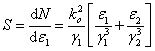(3)

As explained σ does not appear in equation (3). However, MTM's parameter ε2 appears explicitly in the equation. Thus, we can measure the effect of MTM parameters on the sensitivity of our sensor. As well as, we found that the frequency plays important role in the behavior of our sensor.

3. Results and Discussion

To understand the behavior of the propagating fields in the waveguide, we solved the dispersion equation presented in Equation (2) numerically using Maple. The dispersion equation is solved at different values of ε2 and μ2. The numerical values are chosen such that the multiplication ε2 μ2=4. Table 1 presents the selected values of ε2 and μ2. For the dielectric material, ε1 is chosen to be equal 2.25. The Graphene conductivity is taken from  as σ=77.2+j38.6 /i>S.m.

Table 1. Selected values of ε2 and μ2.

 case ε2 μ2 1 -1 -4 2 -4 -1 3 -2 -2 4 -8 -1/2 5 -1/2 -8

Then values of effective refractive index obtained from Equation (2) is used to solve for sensitivity in Equation (3). Figures 2-4 present the obtained results. Figure 2 displays the results obtained for case (2) and case (3) where ε2 values are -4 and -2. Figure 3 shows the results obtained for case (4) where ε2 value -8. In figure 4, results obtained by applying case (1) and case (5) where ε2 values are -1 and -1/2 are exhibited. In all figures we have noticed as the frequency increases the value of sensitivity decreases. This is can be seen from Equation (3) where sensitivity S is proportional to the inverse of the square of the frequency. This may suggest that this sensor is good for the values of frequencies in the range 1-10 THz. It is also clear that as the absolute valued of ε2 increases, the sensitivity increases. This is clearly stated by Equation (3). From Equation (3), we notice that the value of S is directly proportional with ε2.

4. Conclusion

In this paper, we present a new sensor consists of monolayer of Graphene sandwiched between MTM and dielectric. The dispersion equation is derived using Maxwelles'equations. The effective refractive index as function of frequency is numerically solved using Maple. The homogenous sensitivity is derived from the dispersion equation. Then effective refractive index is used to numerically calculate the sensitivity.

Results shows that as ε2 increases the sensitive increase. Also results show that at frequency ranges from 1-10 THz the sensitivity of our sensor decreases dramatically as frequency increases. That conclude that the sensitivity of our proposed senor depends on the MTM parameters and frequency. The dependency of Graphene is shown to be implicit since it doesn't explicitly appears in the sensitivity equation.

The proposed sensor might be used in very sensitive measurements to detect problems in very early stages.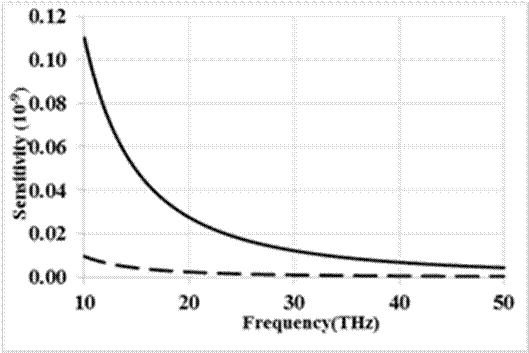Figure 2. The sensitivity as function of frequency. Solid line is using parameters as in case (2). Dashed line is using parameters as in case (3). Sensitivity decreases as frequency increase.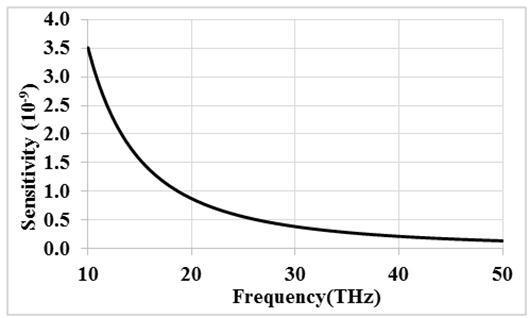Figure 3. The sensitivity as function of frequency using parameters as in case (4). Sensitivity decreases as frequency increase.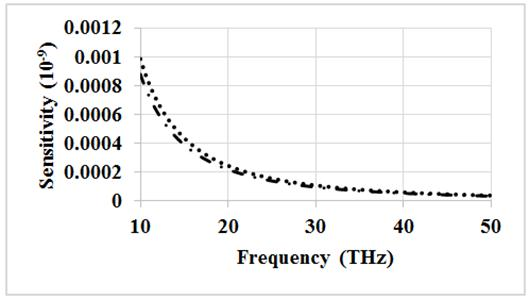Figure 4. The sensitivity as function of frequency. Dotted line is using parameters as in case (1). Dashed-dotted line is using parameters as in case (5). Sensitivity decreases as frequency increase.

References

1. El-Khozondar, H. J. "Hydrostatic Stress Effect on the Optical Performance and the Stress Sensitivity of Optical Nonlinear Waveguide", An - Najah Univ. J. Res. (N. Sc.), 22, 1-16, 2008.
2. El-Khozondar, H. J, El-Khozondar, R. J, Shabat, M. M, Koch, A. W, "Stress Effect on Optical Nonlinear Waveguide Sensor", Journal of optical communication, 28 (3), 175- 179, 2007.
3. Ghatak, A. & Thyagarajan, K, Introduction to Fiber Optics, University Press. Cambridge. U.K, 1998.
4. Cush, R. Cronin, J. Stewart, W. Maule, C. Molloy, J. & Goddard, N, "The resonant mirror: A novel optical biosensor for direct sensing of bio-molecular interactions". Part I: Principle of operation and associated instrumentation, Biosensors Bioelectron. (8). 347-353, 1993.
5. Bernard, A. Bosshard, H, "Real-time monitoring of antigenantibody recognition on a metal oxide surface by an optical grating coupler sensor". European J. Biochem. (230). 416-423, 1995.
6. A. Andryieuski, and A. V. Lavrinenko, Graphene metamaterials based tunable terahertz absorber: effective surface conductivity approach, Opt Express 21: 9144-9155, 2013.
7. H. Tao, N. I. Landy, C. M. Bingham, X. Zhang, R. D. Averitt, and W. J. Padilla, A metamaterial absorber for the terahertz regime: Design, fabrication and characterization, Opt. Soc. Am. 16: 7181-7188, 2008.
8. M. Dragoman, A. A. Muller, D. Dragoman, F. Coccetti and R. Plana, Terahertz antenna based on Graphene, J. Appl. Phys. 107: 104313, 2010.
9. Y. Huang, L. Wu, M. Tang, and J. Mao, Design of a Beam Reconfigurable THz Antenna with Graphene-Based Switchable High-Impedance Surface, IEEE Trans. Nanotechnol. 11: 83642, 2012.
10. J. Chen, M. Badioli, P. Alonso-Gonzalez, S. Thongrattanasiri, F. Huth, J. Osmond, M. Spasenovi c, A. Centeno, A. Pesquera, P. Godignon, A. Z. Elorza, N. Camara, F. J. Garc´a de Abajo, R. Hillenbrand, and F. H. L. Koppens, Optical nano-imaging of gate-tunable Graphene plasmons, Nature (London) 487: 771, 2012.
11. V. G. Veselago, The electrodynamics of substances with simultaneously negative values of ε and μ, Soviet Physics 10: 509-514,1968.
12. D. R. Smith, W. J. Padilla, D. C. Vier, S. C. Nemat-Nasser and S. Schultz, Composite medium with simultaneously negative permeability and permittivity, Phys. Rev. Lett. 84: 4184-4187,2000.
13. H. J. El-Khozondar, R. J. El-Khozondar and S. Zouhdi, Tunable MTMs consists of a single-walled nanotube thin film waveguide covered by nonlinear cladding, Appl. Phys. A 119: 45153, 2015.
14. H. J. El-Khozondar, R. J. El-Khozondar, M. M. Shabat, Double-Negative Metamaterial Optical waveguide Behavior Subjected to Stress, Islamic university journal for natural science and Engineering 16: 9-20, 2008.
15. H. El-Khozondar, R. El-Khozondar, M. Shabat and A. Koch, Metallic nonlinear magnetooptical nonreciprocal isolator, Optik 122: 25658, 2011.
16. H. J. El-Khozondar, M. Abu-Marasa, R. J. El-Khozondar, M. Elbahri, S.Zouhdi, Design of Voltage control Oscillator using Nonlinear Composite Right/Left-Handed Transmission Line, Advanced Electromagnetics 5: 15-21, 2016.
17. M. Jablan, H. Buljan, and M. Soljacic, Plasmonics in Graphene at infrared frequencies, Physical review B 80: 245435, 2009.
18. J. S. G´mez-D´az and J. Perruisseau-Carrier, Propagation of hybrid TM-TE plasmons on magnetically-biased Graphene sheets, Journal of Applied Physics 112: 124906, 2012.
19. X. Gu, Graphene-Based Surface Plasmon-Polaritons for Terahertz Applications, Dissertation, UCLA Electronic Theses and Dissertations, 2013.
20. R. J. El-Khozondar, H. J. El-Khozondar, and M. M. Shabat, "Sensitivity of Graphene-MTM Waveguide ". Fifth Palestinian Conference on Modern Trends in Mathematics and Physics, July 31-August 2, 2016.
21. K. Y. Kim, Y. K. Cho and H. Tae, Guided Modes Propagations of Grounded Double-Positive and Double-Negative Metamaterial Slabs with Arbitrary Material Indexes, Journal of the Korean Physical Society 49: 577-584,2006.
22. X. He, J. Tao, B. Meng, Analysis of Graphene TE surface plasmons in the terahertz regime, Nanotechnol. 24: 345203-345208, 2013.
23. I. P. Kaminow, W. L. Mammel and H. P. Weber, Metal-Clad Optical Waveguides: Analytical and Experimental Study, Apllied Optics 13: 136-405, 1974.
24. F. Wang, Y. Zhang, C. Tian, C. Girit, A. Zettl, M. Crommie and Y. R. Shen, Gate-variable optical transitions in Graphene, Science 320: 206-209, 2008.

 Contents 1. 2. 3. 4.
Article ToolsAbstractPDF(275K)